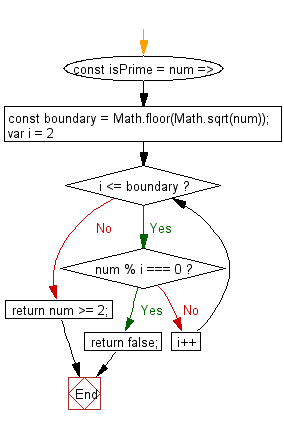# JavaScript: Check whether the provided integer is a prime number or not

## JavaScript fundamental (ES6 Syntax): Exercise-191 with Solution

Write a JavaScript program to check whether the provided integer is a prime number or not.

• Check numbers from 2 to the square root of the given number.
• Return false if any of them divides the given number, else return true, unless the number is less than 2.

Sample Solution:

JavaScript Code:

``````//#Source https://bit.ly/2neWfJ2
const isPrime = num => {
const boundary = Math.floor(Math.sqrt(num));
for (var i = 2; i <= boundary; i++) if (num % i === 0) return false;
return num >= 2;
};

console.log(isPrime(11));
console.log(isPrime(17));
console.log(isPrime(8));
```
```

Sample Output:

```true
true
false
```

Pictorial Presentation:Flowchart:Live Demo:

See the Pen javascript-basic-exercise-191-1 by w3resource (@w3resource) on CodePen.

Improve this sample solution and post your code through Disqus

What is the difficulty level of this exercise?

Test your Programming skills with w3resource's quiz.

﻿

## JavaScript: Tips of the Day

How to check whether a string contains a substring in JavaScript?

ECMAScript 6 introduced String.prototype.includes:

```const string = "foo";
const substring = "oo";

console.log(string.includes(substring));
```

includes doesn't have Internet Explorer support, though. In ECMAScript 5 or older environments, use String.prototype.indexOf, which returns -1 when a substring cannot be found:

```var string = "foo";
var substring = "oo";

console.log(string.indexOf(substring) !== -1);
```

Ref: https://bit.ly/3fFFgZv